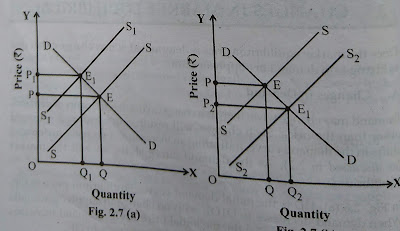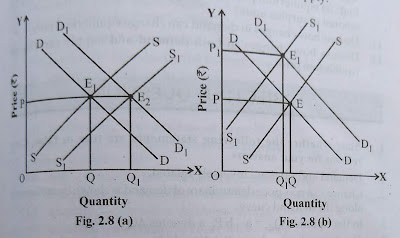# CHANGES IN MARKET EQUILIBRIUM

CHANGES IN MARKET EQUILIBRIUM
Once the market equilibrium price is determined, it may change either due to the demand in nanges or supply or both.

Change in market equilibrium Demand
Demand may change when there is change determines demand other than price such changes in result upward and downward shift in demand curve indicating and increasing or a decrease in demand as demand curve as demand curve shift, so will the market equilibrium.
In Fig 2.6(a) DD is initial Demand Curve. Equilibrium price is OP when demand decreas to D1D1, with no change in supply,price fall's From OP to OP1 In Fig 2.6 the initial price is OP Demand increase From DD to D2D2 with no change in Supply, price rises to OP2Change in market equilibrium Demand
Change market equilibrium in Supply
Supply may change when there is a change in the supply of non-price determinants.  Such changes will result in a decrease or increase in the supply curve moving to the left or to the right, respectively (as discussed in Table 2).  As the supply curve shifts, so will the market equilibrium.
In Fig.  2. 7 (a), the initial equilibrium price is OP.  Supply decreases from SS toSS. With no change in demand, price rises from OP to OP1 and quantity falls from OQ to OQA.  In Fig. 2.7 (b), the initial equilibrium price is OP and quantity is OQ. Whensupply increases from SS to S2 S2 the price falls from OP to OP2 and quantity rises to OQ2.Change market equilibrium in Supply

Change market equilibrium in both Demand and Supply
All time be simultaneous changes in both demand and supply in such as situation in the change equilibrium price of depend upon the relative magnitude of the change in demand and supply.Change market equilibrium in both Demand and Supply
In Fig.  2.8 (a), the initial demand curve is DD and the supply curve is SS.  The equilibrium price is OP. Both demand and supply increase by the same magnitude.  So the price remains the same, but the quantity increases from OQ to OQ1.
In Fig.  2. 8 (b), the original equilibrium price is OP.  Demand increases to D1D1 and supply decreases to S1S1  Asa result price rises from OP to OP1.